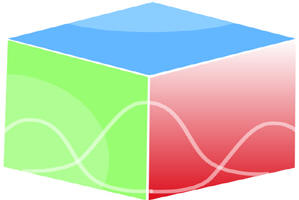``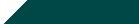Home| Journals | Statistics Online Expert | About Us | Contact Us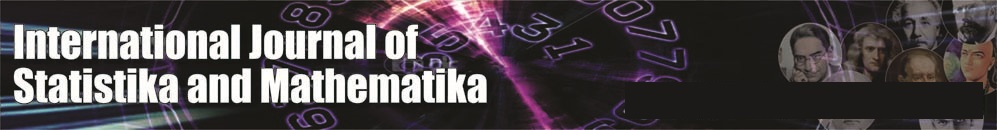Untitled Document

On πgβ-Closed sets in Topological Spaces

A.Devika1, N. Sakthilakshmi2* and R.Vani3

1Associate Professor, Dept. of Mathematics, P.S.G College of Arts and Science, Coimbatore (TN) INDIA.

2Asst.Professor, Dept.Of Maths with CA, Hindusthan College of Arts And Science, Coimbatore (TN) INDIA.

3Asst.Professor, Dept. of Mathematics with CA, P.S.G College of Arts and Science, Coimbatore (TN) INDIA.

[email protected]

Research Article

Abstract: In this paper a new class of sets called πgβ_closed sets is introduced and its properties are studied. Further the notion of πgβ-T½ space and πgβ­-continuity are introduced.

Mathematics Subject Classification: 54A05, 54A40

Keywords: πgβ-closed, πgβ-open, πgβ-continuous, πgβ-T½ spaces.

1. Introduction

Andrijevic  introduced a new class of generalized open sets in a topological space, the so-called β-open sets. This type of sets was discussed by Ekici and Caldas  under the name of γ-open sets. The class of β-open sets is contained in the class of semi-pre-open sets and contains all semi-open sets and preopen sets. The class of β-open sets generates the same topology as the class of preopen sets. Since the advent of these notations, several research paper with interesting results in different respects came to existence ([1,3,6,11,12,21,22,23]).Levine introduced the concept of generalized closed sets in topological space and a class of topological spaces called T½spaces. Extensive research on generalizing closedness was done in recent years as the notions of a generalized closed, generalized semi-closed α-generalized closed, generalized semi -pre-open closed sets were investigated in [2,7,16,18,19].The finite union of regular open sets is said to be π-open. The complement of a π-open set is said to be π-closed.

The aim of this paper is to study the notion of πgβ-closed sets and its various characterizations are given in this paper. In Section 3, the basic properties of πgβ-closed sets are studied. In section 4, the characterize πgβ-open sets is given. Finally in section 5, πgβ-continuous and πgβ-irresolute functions are discussed.

2. Preliminaries

Throughout this paper (X, τ) and (Y, σ) represent non-empty topological spaces on which no separation axioms are assumed unless otherwise mentioned. For a subset A of a space (X, τ) cl (A) and int (A) denote the closure of A and the interior of A respectively. (X, τ) will be replaced by X if there is no chance of confusion.

Let us recall the following definitions which shall be required later.

Definition 2.1: A subset A of a space (X, τ) is called

(1) a pre-open set  if A⊂int (cl (A)) and a preclosed set if cl(int(A))⊂A;

(2) a semi-open set  if A⊂cl (int(A)) and a semi-closed set if int(cl(A))⊂A

(3) a α-open set  if A⊂int(cl(int(A))) and a α-closed set if cl(int(cl(A)))⊂A

(4) a semi-preopen set A⊂cl(intcl(A)) and a semi-pre-closed set if int(cl(int(A)))⊂A

(5) a regular open set if A=int(cl(A)) and a regular closed set if A=cl(int(A));

(6) b-open  or sp-open,γ-openif A⊂cl(int(A))∪int(cl(A)).

The complement of a b-open set is said to be b-closed .The intersection of all b-closed sets of X containing A is called the b-closure of A and is denoted  by bCl(A).The union of all b-open sets of X contained in A is called           b-interior of A and is denoted by bInt(A).The family of all b-open (resp α-open, semi-open,preopen,β-open,            b-closed,preclosed)subsets of a space X is denoted by bO(X)(resp αO(X),SO(X),PO(X),βO(X),bC(X),PC(X)) and the collection of all b-open subsets of X containing a fixed point x is denoted by bO(X,x).

The sets SO(X, x), α(X, x), PO(X, x), βO(X, x) are defined analogously.

Lemma 2.2: Let A be a subset of a space X.Then

(1) bCl(A)=sCl(A)∩pCl(A)=A∪[Int(Cl(A))∩Cl(Int(A))];

(2) bInt(A)=sInt(A)∪pInt(A)=A∩[Int(Cl(A))∪Cl(Int(A))];

Definition 2.3: A subset A of a space (X, τ) is called

(1) a generalized closed (briefly g-closed)  if cl(A)⊂U whenever A⊂U and U is open.

(2) a generalized b-closed (briefly gb-closed)  if bcl(A)⊂U whenever A⊂U and U is open.

(3) πg-closed  if cl (A) ⊂U whenever A⊂U and U is π-open.

(4) πgp-closed  if pcl (A) ⊂U whenever A⊂U and U is π-open.

(5) πgα-closed  if αcl (A) ⊂U whenever A⊂U and U is π-open.

(6) πgsp-closed  if spcl (A) ⊂U whenever A⊂U and U is π-open.

Definition 2.4: A function f: (X, τ) ⟶ (Y, σ) is called

(1) π-irresolute  if f‾ˡ (V) is π -closed in (X, τ) for every π -closed of (Y, σ);

(2) b- irresolute:  if for each b-open set V in Y, f ‾ ˡ (V) is b-open in X:

(3) b-continuous:  if for each open set V in Y, f ‾ ˡ (V) is b-open in X.

3. πgβ-closed sets

Definition 3.1: A is a subset of (X, τ) is called πgβ-closed if βcl (A) ⊂U whenever A⊂U and U is π-open in (X, τ).             BY πGBC (τ) we mean the family of all πgβ-closed subsets of the space (X, τ).

Theorem 3.2:

1. Every closed set is πgβ-closed

2. Every g-closed is πgβ-closed

3. Every α-closed set is πgβ-closed

4. Every pre-closed set is πgβ-closed.

5. Every gβ-closed set is πgβ-closed.

6. Every πg-closed set is πgβ-closed.

7. Every πgp-closed set is πgβ-closed.

8. Every πgα–closed set is πgβ-closed.

9. Every πgs-closed set is πgβ-closed.

10. Every πgβ-closed set is πgsp-closed

Proof: Straight forward converse of the above need not be true as seen in the following examples.

Example3.3:Consider X={a,b,c,d},τ = {ɸ,{a},{d},{a,d},{c,d},{a,c,d},X}.Let A= {c}.Then A is πgβ-closed but not closed, g-closed, α-closed,pre-closed,gβ-closed, πg-closed.

Example 3.4:Let X ={a,b,c,d,e} and τ ={ɸ ,{a,b},{c,d},{a,b,c,d},X}.Let A ={a}.Therefore A is πgβ-closed but not πgα-closed.

Example3.5: Let X={a,b,c,d,e} and τ = {ɸ ,{a,b},{c,d},{a,b,c,d},X}.

Let A = {a, b}.Therefore A is πgβ-closed but not πgp-closed.

Rewmark 3.6: The above discussions are summarized in the following diagram.

Closed set                               g-closed set                          αg-closed                                gp-closed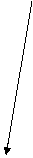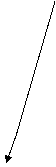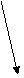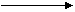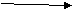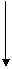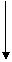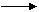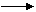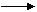α-closed  gα-closed gs-closed                πg-closed               πgα- closed                πgp-closed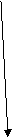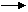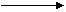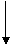Semi closed set  sg-closed set               gsp-closed set         πgβ-closed set           πgsp-closed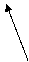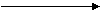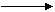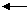semi pre closed set          pre closed                    β-closed                                   gβ-closed

Theorem3.7: If A is π-open and πgβ-closed, then A is β-closed.

Proof: Let A is π-open and πgβ-closed.Let A⊂A where A is π-open. Since A is πgβ-closed, βcl (A) ⊂A.Then

A=βcl (A).Hence A is β-closed.

Theorem 3.8: Let A be a πgβ-closed in (X, τ).Then βcl (A)-A does not contain any non empty π-closed set.

Proof:Let F be a non empty π-closed set such that F⊂βcl(A)-A.since A is πgβ closed,A⊂X-F where X-F is π-open implies βcl(A)⊂X-F.Hence F⊂X- βcl(A).Now F⊂βcl(A)∩(X-βcl(A)) implies F=ɸ which is a contradiction. Therefore βcl (A) does not contain any non empty π–closed set.

Corollary3.9: Let A be πgβ-closed in (X, τ).Then A is β-closed iff βcl (A)-A is π- closed.

Proof: Let A be β-closed. Then βcl (A) =A. This implies βcl (A)-A=ɸ which is π-closed. Assume βcl (A)-A is           π-closed. Then βcl (A)-A=ɸ. Hence βcl (A) =A.

Remark 3.10: Finite union of πgβ-closed sets need not be πgβ-closed.

Example 3.11: Consider X={a,b,c}, τ ={ ɸ ,{a},{b},{a,b},X}.Let A = {a},B={b}.Here A and B are πgβ-closed but A∪B ={a,b} is not πgβ-closed.

Remark 3.12: Finite intersection of πgβ-closed sets need not be πgβ-closed.

Example3.13:Consider X ={a,b,c,d},τ ={ ɸ,{a},{b},{a,b},{a,b.c},{a,b,d},X}.Let A= {a,b,c},B={a,b,d}.Here A and B are πgβ-closed but A∩B ={a,b} is not πgβ-closed.

Definition3.14 : Let (X, τ) be a topological space A⊂X and xϵX is said to be β-limit point of A iff every β-open set containing x contains a point of A different from x.

Definition 3.15: Let (X,τ ) be a topological space,A⊂X.The set of all β-limit points of A is said to be β-derived set of A and is denoted by Db [A]

Lemma 3.16 : If D (A) = Db [A], then we have cl (A) = βcl (A)

Lemma3.17 : If D (A) ⊂Db [A] for every subset A of X.Then for any subsets F and B of X, we have

Βcl (F∪B) = βcl(F)∪βcl(B)

Theorem3.18: Let A and B be πgβ-closed sets in (X, τ) such that D [A] ⊂Db [A] and D [B] ⊂Db [B]

Then A∪B is πgβ-closed.

Proof: Let U be π–open set such that A∪B⊂U.Since A and B are πgβ-closed sets we have β cl(A)⊂U and βcl(B)⊂U.Since D[A]⊂Db [A] and D[B]⊂Db [B] ,by lemma 3.16,cl(A)=βcl(A) and cl(B)=βcl(B).Thus

βcl(A∪B)⊂cl (A∪B)=cl(A)∪cl(B)=βcl(A)∪βcl(B)⊂U.This implies A∪B is πgβ-closed.

Theorem3.19: If A is πgβ-closed set and B is any set such that A⊂B⊂βcl (A), then B is πgβ-closed set.

Proof: Let B⊂U and U be π-open. Given A⊂B.Then A⊂USince A is πgβ-closed, A⊂U implies βcl (A) ⊂U.By assumption it follows that  βcl(B)⊂βcl(A)⊂U.Hence B is a πgβ-closed set.

4. πgβ-open sets

Definition 4.1: A set A⊂X is called πgβ-open if and only if its complement is πgβ-closed.

Remark4.2: βcl(X-A) =X-βint (A)

By πGBO (τ) we mean the family of all πgβ-open subsets of the space (X, τ).

Theorem4.3: If A⊂X is πgβ-open iff F⊂βint (A) whenever F is π-closed and F⊂A

Proof: Necessity: Let A be πgβ-open.Let F be π-closed and F⊂A.Then X-A⊂X-F where X-F is π-open. By assumption, βcl(X-A) ⊂X-F.By remark 4.2, X- β int (A) ⊂X-F.Thus F⊂βint (A).

Sufficiency: Suppose F is π-closed and F⊂A such that F⊂βint (A).Let X-A⊂U where U is π -open. Then X-U⊂A when X-U is π-closed. By hypothesis, X-U⊂β int (A).X-β int (A) ⊂U. βcl(X-A) ⊂U.Thus X-A is πgβ-closed and A is πgβ-open.

Theorem4.4: If βint (A) ⊂B⊂A and A is πgβ-open then B is πgβ-open.

Proof: Let βint (A) ⊂B⊂A.Thus X-A⊂X-B⊂βcl(X-A).Since X-A is πgβ-closed, by theorem 3.19, (X-A) ⊂(X-B) ⊂βcl(X-A) implies (X-B) is πgβ-closed.

Remark 4.5: For any A⊂X, βint (β cl (A)-A) = ɸ

Theorem 4.6: If A⊂X is πgβ-closed, then βcl (A)-A is πgβ-open.

Proof: Let A be πgβ-closed let F be π–closed set.F⊂βcl (A)-A. By theorem3.8, F=ɸ .By remark4.5,

βint (β (clA)-A) =ɸ .Thus F⊂βint (βcl (A)-A).Thus βcl (A)-A is πgβ-open.

Lemma4.7 : Let A⊂X.If A is open or dense, then πO (A, τ /A) =V ∩ A such that V ϵ π O(X, τ).

Theorem 4.8: Let B ⊂ A ⊂ X where A is πgβ-closed and π-open set. Then B is πgβ-closed relative to A iff B is

Πgβ-closed in X.

Proof: Let B⊂A ⊂ X.Where A is πgβ-closed and π-open set. Let B be πgβ-closed in A.Let B⊂U where U is π-open in X.Since B⊂A, B=B∩A⊂U∩A, this implies βcl(B) =βclA (B)⊂U∩A⊂U.Hence, B is πgβ -closed in X.

Let B be πgβ-closed in X.Let B⊂O where O is π-open in A.Then O=U∩A where U is π-open in X.This implies    B⊂ O=U ∩ A ⊂ U.Since B is π g β –closed in X, βcl (B) ⊂U.Thus βclA (B) =A∩β cl(B)⊂U A =O.Hence,B is     πgβ-closed relative to A.

Corollary 4.9: Let A be π-open, πgβ-closed set. Then A∩F is πgβ-closed whenever FϵβC(X).

Proof: Since A is πgβ-closed and π-open, then βcl (A) ⊂A and thus A is β-closed. Hence A∩F is β-closed in X which implies A∩F is πgβ-closed in X.

Definition 4.10: A space (X,τ ) is called a πgβ-T1/2 space if every πgβ-closed set is β-closed.

Theorem 4.11:

(i)BO (τ) ⊂πGBO (τ)

(ii) A space (X, τ) is πgβ-T1/2 iff BO (τ) =πGBO (τ).

Proof :( i) Let A be β-open, then X-A is β-closed so X-A is πgβ-closed.Thus A is πgβ-open.Hence

BO (τ) ⊂πGBO (τ)

(ii)Necessity: Let (X, τ) be πgβ-T1/2 space. Let AϵπGBO (τ).Then X-A is πgβ-closed.By hypothesis-A is β-closed thus AϵBO (τ).Thus πGBO (τ) =BO (τ).

Suffiency: Let BO (Τ) =πGBO (τ).Let A be πgβ-closed.Then X-A is πgβ-open X-AϵπGBO (τ).X-AϵBO (τ).Hence A is β-closed. This implies (X, τ) is πgβ-T1/2 space.

Lemma 4.12: Let A be a subset of (X, τ) and xϵX.Then xϵβcl (A) iff V∩ɸ for every β-open set V containing x.

Theorem 4.13: For a topological space (X, τ) the following are equivalent

(i)X is πgβ-T1/2 space.

(ii) Every singleton set is either π-closed or β-open.

Proof: To prove (i) ⇒ (ii): Let X be a πgβ-T1/2 space. Let xϵX and assuming that {x} is not π-closed. Then clearly X-{x} is not π-open. Hence X-{x} is trivially a πgβ-closed.Since X is πgβ-T1/2 space-{x} is β-closed. Therefore {x} is β –open.

(ii) ⇒ (i): Assume every singleton of X is either π-closed or β-open. Let A be a πgβ-closed set. Let {x} ϵ β cl (A).

Case (i): Let {x} be π closed. Suppose {x} does not belong to A. Then {x} ϵ β cl (A)-A.By theorem3.8, {x} ϵ A. Hence β cl (A) ⊂ A.

Case (ii): Let {x} be β-open. Since {x} ϵ β cl (A), we have {x} ∩ A ≠ɸ ⇒ {x} ϵ A. Therefore β cl (A) ⊂ A. Therefore A is β-closed.

5. πgβ-continuous and πgβ-irresolute functions

Definition 5.1:A function f:(X,τ )→(Y,σ ) is called πgβ-continuous if every f ‾ 1  (V) is πgβ-closed in (X,τ ) for every closed set V of (Y,σ ).

Definition 5.2:A function f(X,τ)⟶(y,σ )is called πgβ-irresolute if  f ‾ 1 (V) is πgβ-closed in (X,τ ) for every          πgβ- closed set V in  (Y,σ )

Proposition 5.3: Every πgβ-irresolute function is πgβ-continuous.

Remark 5.4: Converse of the above need not be true.

Proof: Let f :( X, τ) ⟶ (Y, σ) be a πgβ-continuous function and V be a πgβ-closed in (Y, σ).

But every πgβ-closed set need not be closed in (Y, σ).So there exists some sets which is not closed in (Y, σ).By definition there exits some sets which are not πgβ-closed in (X, τ), which implies f is not πgβ-irresolute.

Remark 5.6: Composition of two πgβ-continuous functions need not be πgβ-continuous.

Proof: Let f :( X τ) ⟶ (X, σ) and g :( X, σ) ⟶ (X, η) be two πgβ-continuous functions.

Let V be a closed set in (X, η).Since g is a πgβ-continuous function, g‾ 1 (V) is πgβ-closed set in (X, σ).But every πgβ-closed is not closed. Therefore there exist some sets in (X, σ) which is not πgβ-closed in (X, τ).

Hence g₀f is not πgβ-continuous.

Definition5.8: A function f: X ⟶ Y is said to be pre β-closed if f (U) is β-closed in Y for each β-closed set in X.

Proposition 5.9: Let f :(X,τ )→(Y,σ ) be π-irresolute and pre β-closed map. Then f (A) is πgβ-closed in Y for every πgβ-closed set A of X.

Proof: Let A be πgβ–closed in X.Let f(A)⊂V where V is π-open in Y.Then A⊂ f ‾ 1  (V) and A is πgβ–closed in X implies βcl(A)⊂f ‾ 1 (V).Hence f(β cl(A))⊂V.Since f is pre β-closed, βcl(A)⊂βcl(f(βcl(A)))=f(βcl(A))⊂V.Hence f(A) is πgβ-closed in Y.

Definition 5.10: A topological space X is a πgβ-space if every πgβ-closed set is closed.

Proposition 5.11: Every πgβ space is πgβ-T1/2 space.

Theorem 5.12: Let f :(X,τ ) ⟶ (Y,σ ) be a function.

(1)If is πgβ-irresolute and X is πgβ- T1/2 space, then f is β-irresolute.

(2)If f is πgβ-continuous and X is πgβ-T1/2 space, then f is β-continuous.

Proof :( 1) Let V be β-closed in Y.Since f is πgβ-irresolute, f ‾ 1 (V) is πgβ-closed in X.Since X is πgβ-T1/2 space,        f ‾ 1 (V) is β-closed in X.Hence f is β-irresolute.

(2)Let V be closed in Y.Since f is πgβ continuous, f ‾ 1 (V) is πgβ-closed in X.By assumption, it is β-closed. Therefore f is β-continuous.

Definition5.13 : A function f: (X,τ ) ⟶ (Y,σ ) is π-open  map in Y for every π-open in X

Theorem5.14: If the bijective f: (X,τ ) ⟶ (Y,σ ) is β-irresolute and π-open map, then f is πgβ-irresolute.

Proof: Let V be πgβ-closed in Y.Let f ‾ 1 (V)⊂U where U is π-open in X.Then V ⊂ f(U)and f(U)is  π-open implies βcl(V)⊂f(U).Since f is β-irresolute,(f‾1(βcl(V))) is β-closed. Hence βcl(f‾1 (V))⊂βcl(f‾1(βcl(V)))=f‾1(βcl(V))⊂U. Therefore is πgβ-irresolute.

Theorem 5.15: If f: X⟶Y is π-open, β-irresolute, pre β-closed subjective function. If X is πgβ-T1/2 space, then Y is πgβ-T1/2 space.

Proof: Let V be πgβ-closed in Y.Let f ‾ 1 (V)⊂U where U is π-open in X.Then F⊂f(U) and F is a πgβ–closed set in Y implies β cl(F)⊂f(U).Since f is β-irresolute, β cl( f ‾ 1 (F))⊂β cl(f ‾ 1β  cl(F)))= f ‾ 1(β cl(F)) ⊂ U.Therefore             f ‾ 1 (F) = F is β-closed in Y.Hence Y is πgβ-T1/2 space.

References

1. D.Andrijevic, Semipreopen sets, Mat.Vesnik., 38;24-32, 1986.
2. S.P.Arya and T.M.Nour, Characterizations of s-normal spaces, Indian J.Pure Appl.Math., 21; no.8, 717-719, 1990.
3. D.Andrijevic, On β-open sets, Mat.Vesnik 48; 59-64, 1996.
4. G.Aslim, A.Caksu Guler and T.Noiri, on πgs-closed sets in topological Spaces, Acta Math.Hungar., 112(4); 275-283, 2006.
5. Ahmad-Al-Omari and Mohd.Salmi Md Noorani, On Generalized β –closed Sets, Bull.Malays.Math.Sci.Soc., 32(1); 19-30, 2009.
6. M.Caldas and S.Jafari.On some applications of β-open set in topological spaces, Kochi J.Math., 2;11-19, 2007.
7. J.Dontchev, On generalizing semi-preopen sets, Mem.Fac.Kochi Univ.Ser.A Math., 16; 35-48, 1995.
8. J.Dontchev and M.Przemski, On the various decompositions of continuous and some weakly continuous functions, Acta Math.Hungar.71; 109-120, 1996.
9. J.Dontchevand M.Ganster, Onδ-generalized closed sets and T¾-spaces, Mem.Fac.Kochi Univ.Ser.A Math., 17; 15-31, 1996.
10. J.Dontchev and T.Noiri,Quasi Normal Spaces and π g-closed sets,Acta Math.Hungar.,89(3); 211-219, 2000.
11. E.Ekici and M.Caldas, Slightly-continuous functions, Bol.Soc.Parana.Mat. (3) 22; 63-74, 2004.
12. M.Ganster and M.Steiner, On some questions about β –open sets, Questions Answers Gen.Topology 25; 45-52, 2007.
13. M. Ganster and M.Steiner, On b τ-closed sets, Appl. Gen.Topology 8; 243-247, 2007.
14. C. Janaki, Studies on πgα-closed sets in Topology, Ph.D Thesis, Bharathiar University, Coimbatore, 2009.
15. N. Levine, Semi-open sets and semi-continuity in topological spaces, Amer.Math.Monthly70; 36-41, 1963.
16. N. Levine, Generalized closed sets in Topology, Rend.Circ.Mat.Palermo (2)19; 89-96, 1970.
17. A.S Mashhour, M.E.Abd El-Monsef and S.N.El-Deep, On pre continuous and weak pre-continuous mappings, Proc.Math.Phys.Soc.Egypt No.53; 47-53, 1982.
18. H.Maki, R.Devi and K.Balachandran, Associated topologies of generalized α-closed sets and α-generalized closed sets, Mem.Fac.Sci.Kochi Univ.Ser.A Math.15; 51-63, 1994.
19. H.Maki, J.Umehara and T.Noiri, every topological space is pre-T T1/2, Mem.Fac.Sci.Kochi Univ. Ser. A. Math., 17; 33-42, 1996.
20. O. Njastad, On some classes of nearly open sets, Pacific J.Math.15; 961-970, 1965.
21. A. A. Nasef, On b-locally closed sets and related topics, Chaos Solitons Fractals 12; 1909-1915, 2001.
22. A .A. Nasef, On Some properties of contra-continuous functions, Chaos Solitons Fractals 24; 471-477, 2005.
23. J. H. Park, Strongly θ-b-continuous functions, Acta Math.Hungar.110; 347-359, 2006.
24. J. H. Park, On πgp-closed sets in Topological Spaces, Indian.J.Pure Appl.Math., (To appear).
25. M. S. Sarsak and N. Rajesh, π-Generalized semi-preclosed Sets, International Mathematical Forum, 5;573-578, 2010.